## 用户运营：RFM分层实战演练

18 评论 1.1万 浏览 167 收藏 7 分钟1. 通过用户的成长轨迹，根据用户的生命周期做定义：导入期-成长期-成熟悉-休眠期-流失期，每个阶段对用户的运营目标都不一样。
2. 根据用户的关键行为做划分，对用户进行区隔，实现用户分层。• R 最近一次交易的时间：R值最大，说明发生交易的时间越久，反之，发生交易的时间越近。
• F 交易的频次：F越大，说明交易频繁，是平台的用户用户，反之，用户不够活跃。
• M 交易的总金额：M越大，客户价值越高，是平台的重点用户。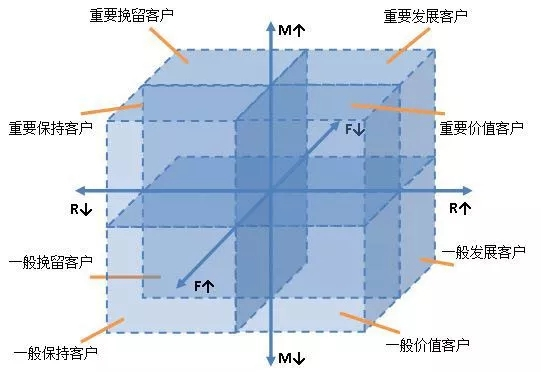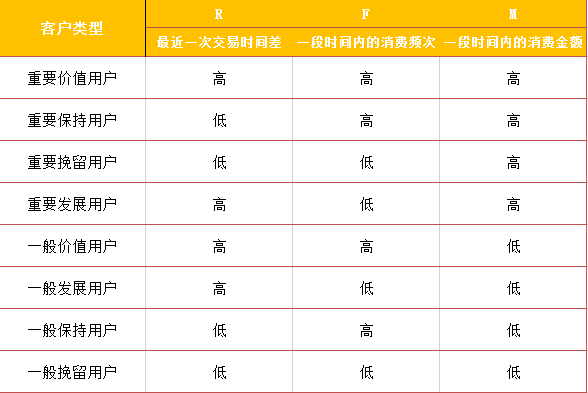## 二、RFM模型的运用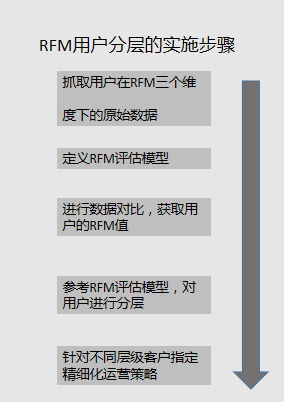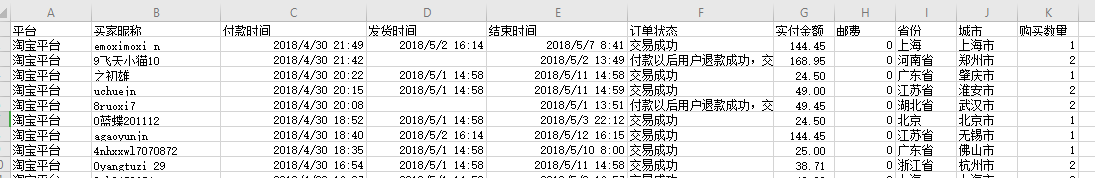### 2. 定义RFM评估模型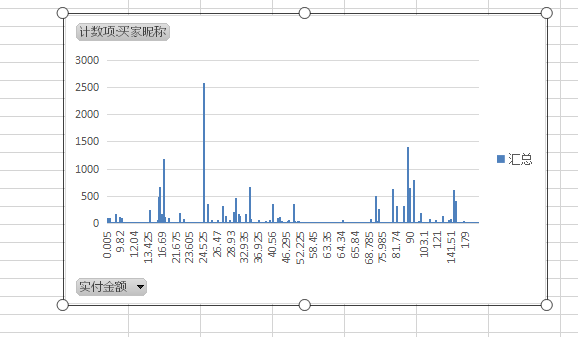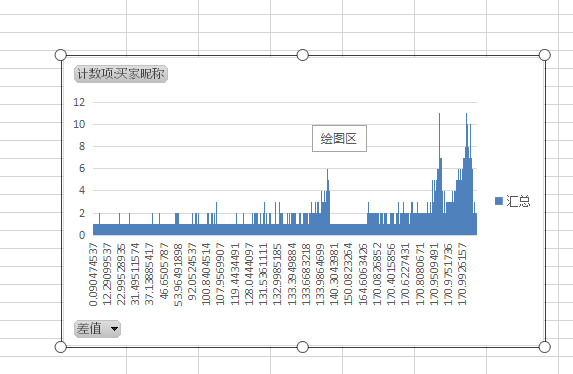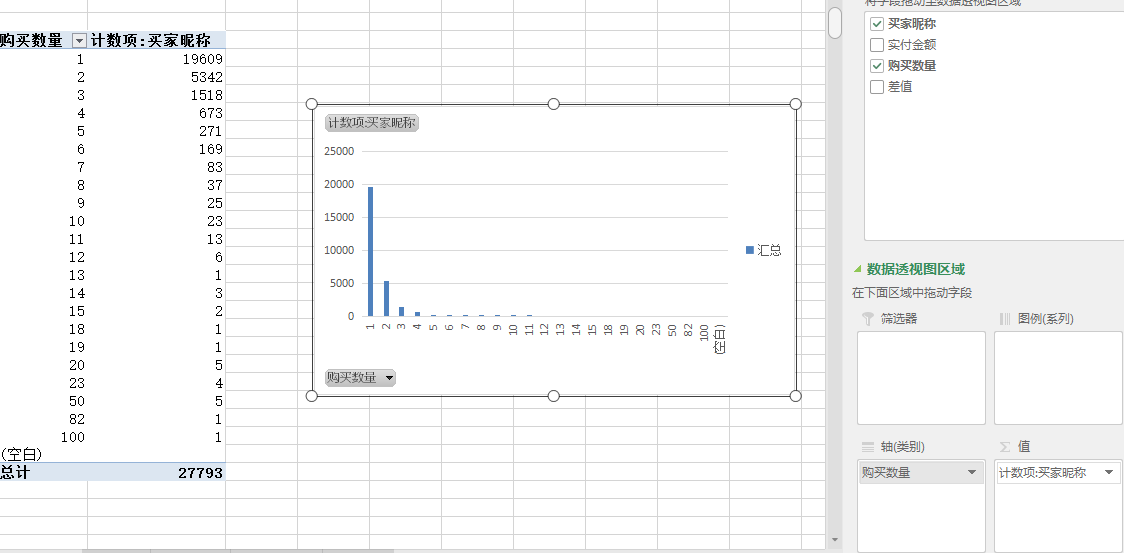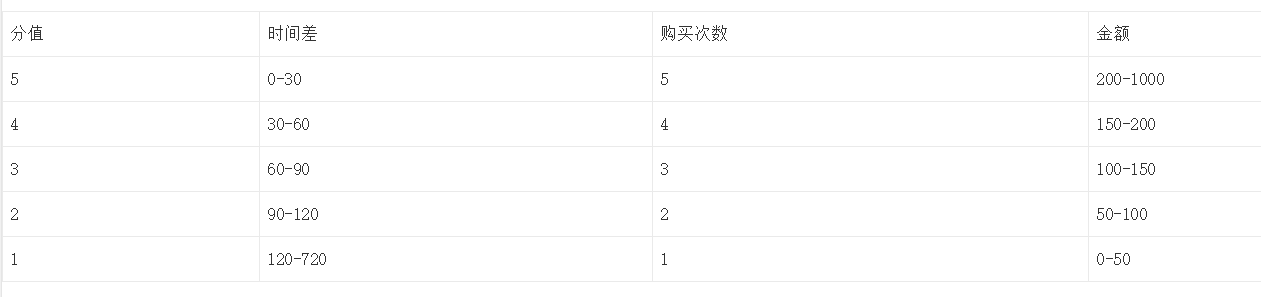### 3. 先算出R值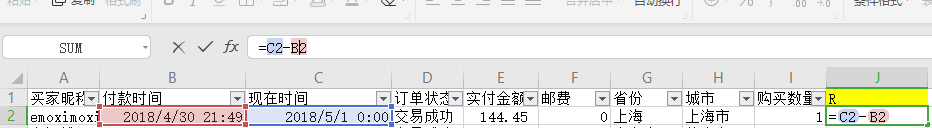`IF(D10<=30,5,IF(D10<=60,4,IF(D10<=90,3,IF(D10<=120,2,1))))`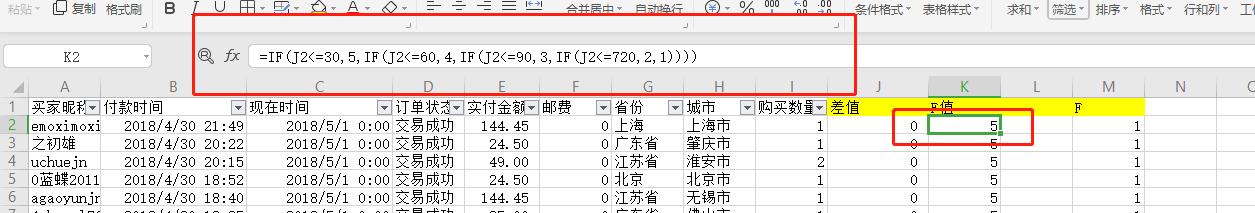### 4. 算出F值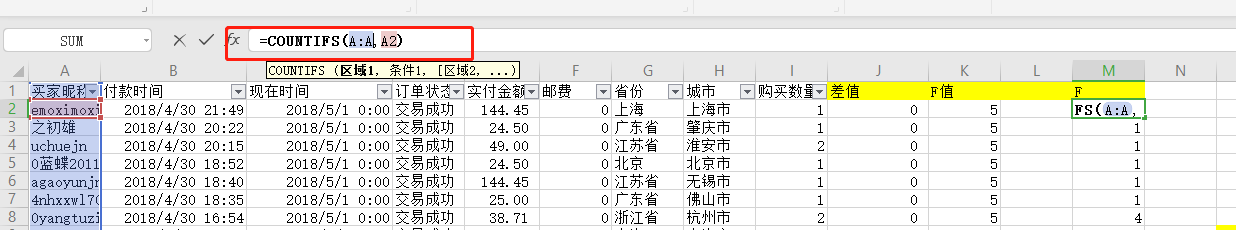### 5. 算出M值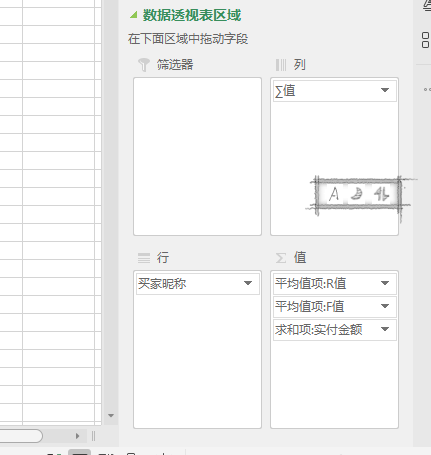### 6. 进一步计算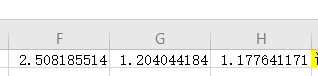`IF(B6>\$F\$1,"高","低")`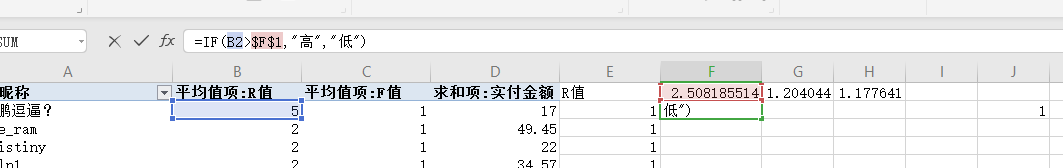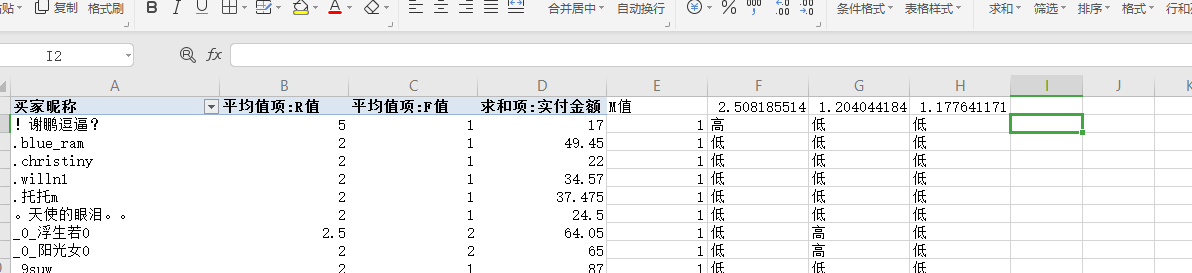### 7. 用if函数自动计算出用户层级

```IF(AND(F2="高",G2="高",H2="高"),"重要价值用户",
IF(AND(F2="高",G2="低",H2="高"),"重要保持用户",
IF(AND(F2="低",G2="高",H2="高"),"重要发展用户",
IF(AND(F2="低",G2="低",H2="高"),"重要挽留用户",
IF(AND(F2="高",G2="高",H2="低"),"一般价值用户",
IF(AND(F2="高",G2="低",H2="低"),"一般发展用户",
IF(AND(F2="低",G2="高",H2="低"),"一般保持用户",
IF(AND(F2="低",G2="低",H2="低"),"一般挽留用户"))))))))```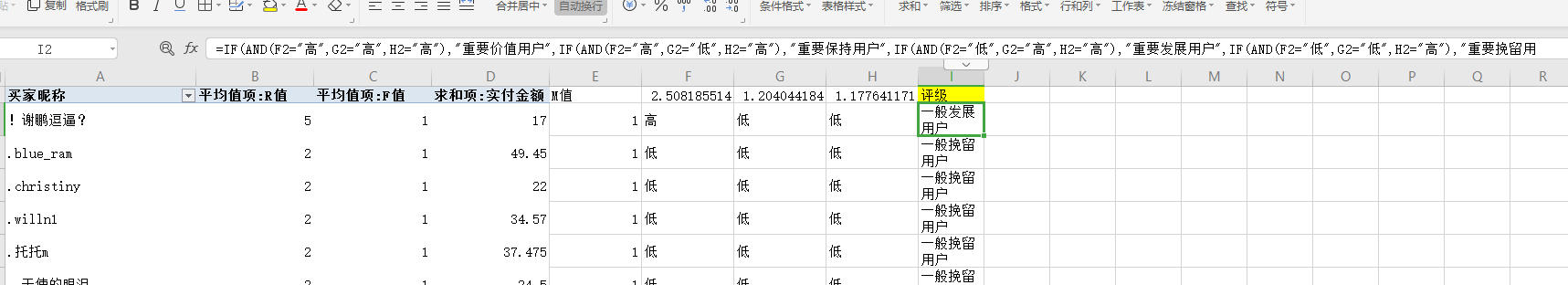### 8. 做表，制定策略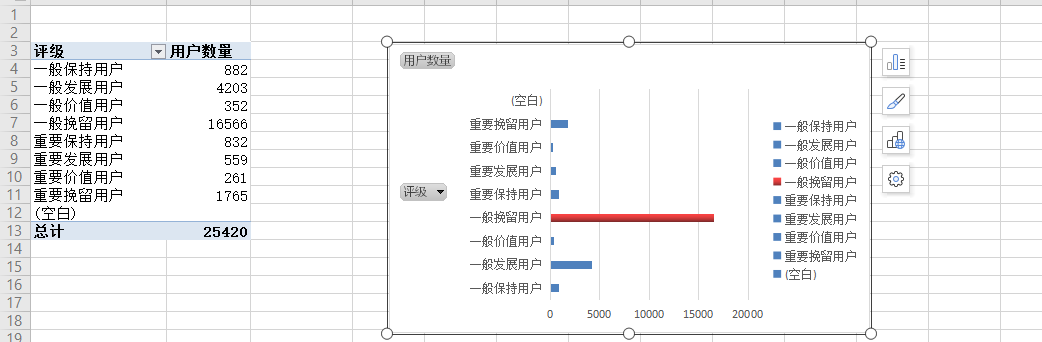1. 制定什么策略呢

回复
2. 你这套算法是有问题，看似还很“科学”的用了个平均值作为“高”和“低”的切割。但是你第一步就错了。你根据什么逻辑来切割R\F\M的1~5分对应的区间的。
你在这一步非常一厢情愿，所以最终基于所谓的“平均值”来分的高低也都是一厢情愿。

回复
3. 每个值分层5个层级的意义何在，你操作用的是每个值分成高低2个层级。

回复
4. 运营经理

回复
5. 有所启发

回复
6. 作家有步骤没懂，可否微信问问

回复
1. 小白一枚，可以加一下微信问问嘛？有一个问题可以咨询一下吗？

回复
2. 你留微信我加你

回复
7. 有错别字吗？

回复
8. 是否应该在定义高或低的时候 把二八法则考虑进去，都不是取均值

回复
1. 这里的中值指的是平均值还是中位数呀？我觉得这里如果也用二八分的话被判断为高的一定非常高，但被判断为低的不一定是真的低，可能只是普通或不是特别高

回复
2. 应该是平均数，中位数的小数点后就只可能是0或者5了，不会有这么长一串小数

回复
3. 额嗯 考虑的是阈值的合理性这个

回复
9. 请问可以告诉我一下数据是从哪里收集的吗？我需要一些数据撰写关于RFM模型的论文，现在收集不到数据？谢谢

回复
10. 已阅，感谢分享！

回复
11. 不是很清楚三个维度如何划分成5个层级，每个层级的值是怎么定义的？

回复
1. 自己去把3个纬度按照(a,b,c) 看看可以产生多少中结果，或者你可以用十进制去表示。000代表3个纬度都低，010 100 101 还有不明白的吗

回复
2. 也不是很明白 😥

回复